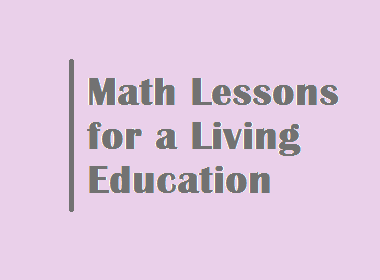# Math Lessons for a Living Education## Math Lessons for a Living Education

Math is a fundamental skill that is essential for success in school, work, and life in general. However, many students struggle with math. This is often because math is taught in a way that is abstract and disconnected from the real world.

A living education approach to math teaching focuses on making math meaningful and relevant to students' lives. Students learn math concepts through hands-on activities, real-world applications, and stories.

Here are some tips for teaching math using a living education approach:

·      Start with concrete examples. Before introducing a new math concept, start with concrete examples that students can relate to. For example, if you are teaching about addition, you could start by showing students two groups of blocks and asking them how many blocks there are in total.

·      Use hands-on activities. Hands-on activities are a great way for students to learn math concepts in a concrete way. For example, you could have students use manipulatives such as blocks or counters to learn about addition and subtraction.

·      Make it real-world. Connect math concepts to the real world whenever possible. For example, if you are teaching about multiplication, you could show students how to calculate the cost of items at a grocery store.

·      Use stories. Stories are a great way to make math more engaging and memorable for students. For example, you could tell a story about a character who is trying to solve a math problem.

Here are some specific examples of math lessons that you can teach using a living education approach:

·      Addition: To teach addition to young children, you can start by showing them two groups of objects and asking them how many objects there are in total. You can also use manipulatives such as blocks or counters to help students visualize the addition process. For example, you could give each student a group of blocks and ask them to add another group of blocks. Then, have the students count the total number of blocks.

·      Subtraction: To teach subtraction to young children, you can start by showing them a group of objects and then taking some of the objects away. Ask the students how many objects are left. You can also use manipulatives such as blocks or counters to help students visualize the subtraction process. For example, you could give each student a group of blocks and ask them to take away some of the blocks. Then, have the students count the total number of blocks that are left.

·      Multiplication: To teach multiplication to young children, you can start by showing them groups of objects and asking them how many objects are in each group. Then, ask the students how many objects there are in total. You can also use manipulatives such as blocks or counters to help students visualize the multiplication process. For example, you could give each student a group of blocks and ask them to multiply the number of blocks in their group by another number. Then, have the students count the total number of blocks.

·      Division: To teach division to young children, you can start by showing them a group of objects and asking them to divide the group into smaller groups. You can also use manipulatives such as blocks or counters to help students visualize the division process. For example, you could give each student a group of blocks and ask them to divide the group into two smaller groups. Then, have the students count the number of blocks in each smaller group.

As students get older, you can introduce more complex math concepts using the same living education approach. For example, you could teach students about fractions, decimals, percentages, and algebra using concrete examples, hands-on activities, real-world applications, and stories.

Here are some additional tips for teaching math using a living education approach:

·      Make math fun. Math should be enjoyable for students. If students are not having fun, they are less likely to learn. You can make math fun by using games, activities, and stories.

·      Create a positive learning environment. Students learn best in a positive and supportive learning environment. Encourage students to ask questions and to help each other.

·      Be patient and persistent. Learning math takes time and practice. Don't get discouraged if students don't understand a concept right away. Just keep practicing and eventually, they will get it.

A living education approach to math teaching can help students to develop a deep understanding of math concepts and to learn to apply math to the real world. By following the tips above, you can create a positive and supportive learning environment where students can thrive in math.